Question

# Test the claim that the mean GPA of night students is smaller than 2.3 at the...

Test the claim that the mean GPA of night students is smaller than 2.3 at the 0.025 significance level.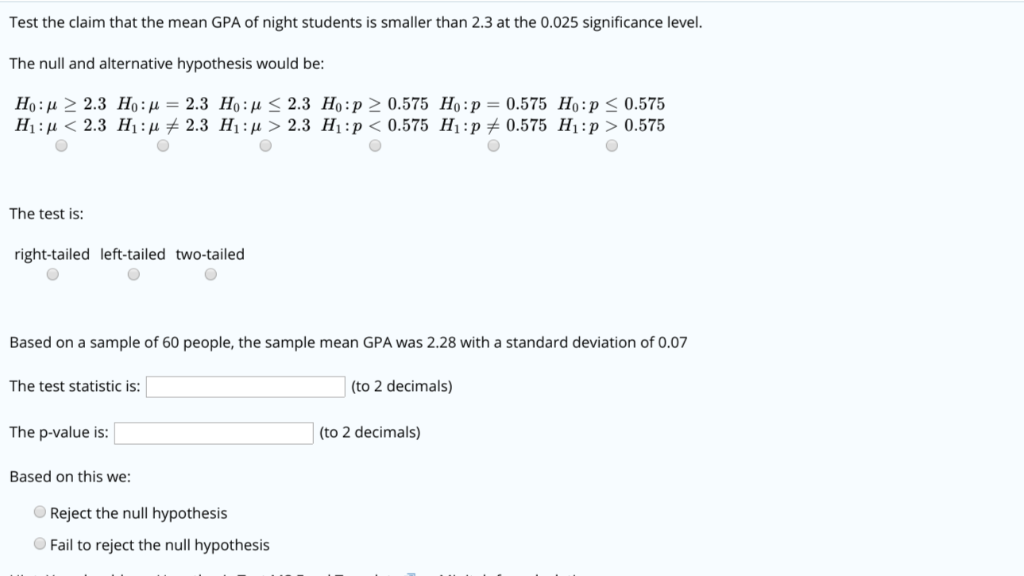Test the claim that the mean GPA of night students is smaller than 2.3 at the 0.025 significance level The null and alternative hypothesis would be: Ho:2 2.3 Ho:H 2.3 Ho: 2.3 Ho:p 2 0.575 Ho:p 0.575 Ho:p0.575 H1: μ < 2.3 H1: μメ2.3 H1ιμ > 2.3 Hi : p < 0.575 H1: pチ0.575 Hi :p > 0.575 The test is: right-tailed left-tailed two-tailed Based on a sample of 60 people, the sample mean GPA was 2.28 with a standard deviation of 0.07 The test statistic is The p-value is: Based on this we: (to 2 decimals) (to 2 decimals) Reject the null hypothesis Fail to reject the null hypothesis

Solution :

This is the left tailed test .

The null and alternative hypothesis is ,

H0 :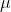= 2.3

Ha :< 2.3

Test statistic = z

= (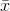-) /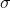/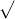n

= (2.28 - 2.3) / 0.07 /60

Test statistic = -2.21

P(z < -2.21) = 0.0136

P-value = 0.01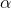= 0.025

P-value <Reject the null hypothesis .

#### Earn Coins

Coins can be redeemed for fabulous gifts.

Similar Homework Help Questions
• ### Test the claim that the mean GPA of night students is smaller than 2.8 at the...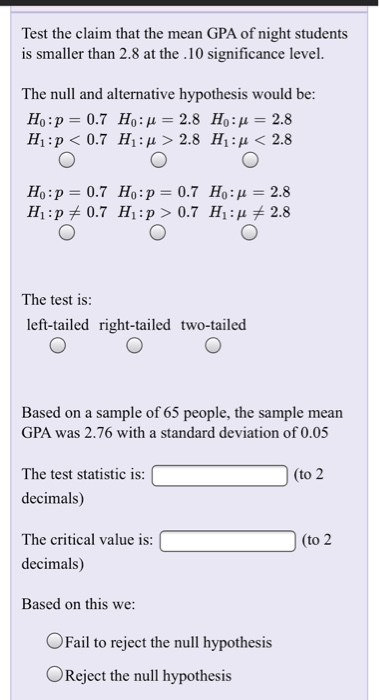Test the claim that the mean GPA of night students is smaller than 2.8 at the .10 significance level. The null and alternative hypothesis would be: H1 : p < 0.7 H1ιμ>2.8 H1 : μ < 2.8 Ho:p 0.7 Ho:p 0.7 Ho: 2.8 The test is: left-tailed right-tailed two-tailed Based on a sample of 65 people, the sample mean GPA was 2.76 with a standard deviation of 0.05 The test statistic is: decimals) The critical value is: decimals) Based on...

• ### Test the claim that the mean GPA of night students is smaller than 2.3 at the....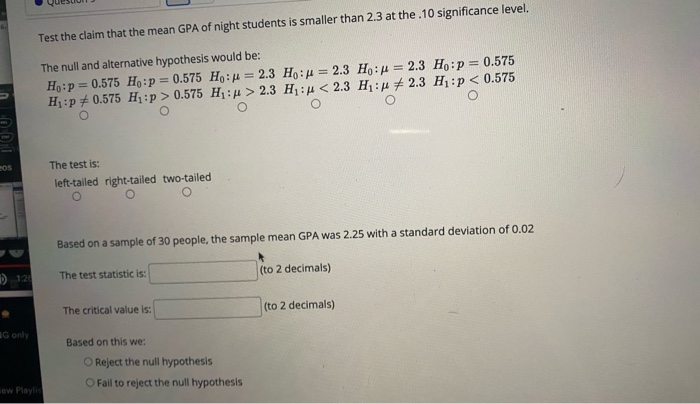Test the claim that the mean GPA of night students is smaller than 2.3 at the. 10 significance level. The null and alternative hypothesis would be: Ho:p = 0.575 H :p = 0.575 Hou = 2.3 Horn = 2.3 H: = 2.3 Ho: p = 0.575 H: p0.575 H:p > 0.575 H: > 2.3 H: 4 < 2.3 H: *2.3 Hp < 0.575 eos The test is: left-tailed right-tailed two-tailed Based on a sample of 30 people, the sample mean...

• ### Test the claim that the mean GPA of night students is significantly different than 2.3 at...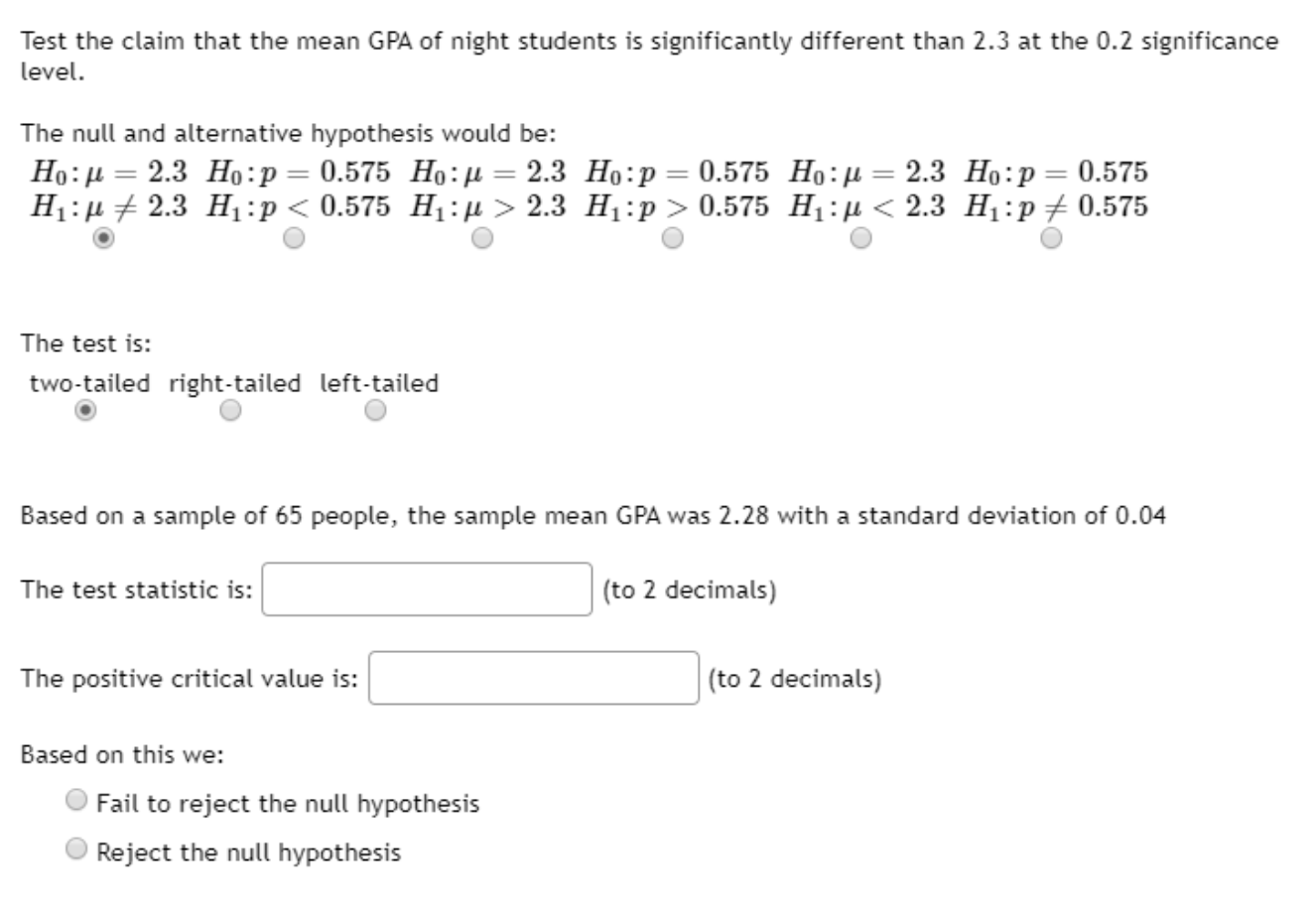Test the claim that the mean GPA of night students is significantly different than 2.3 at the 0.2 significance level. The null and alternative hypothesis would be: Ho:= 2.3 Ho:p = 0.575 Ho:f = 2.3 Ho:p = 0.575 H.:= 2.3 Ho:p=0.575 H:H+ 2.3 H :p < 0.575 H : > 2.3 H:p > 0.575 H:4 < 2.3 H :P + 0.575 The test is: two-tailed right-tailed left-tailed Based on a sample of 65 people, the sample mean GPA was 2.28...

• ### Test the claim that the mean GPA of night students is smaller than 3.2 at the...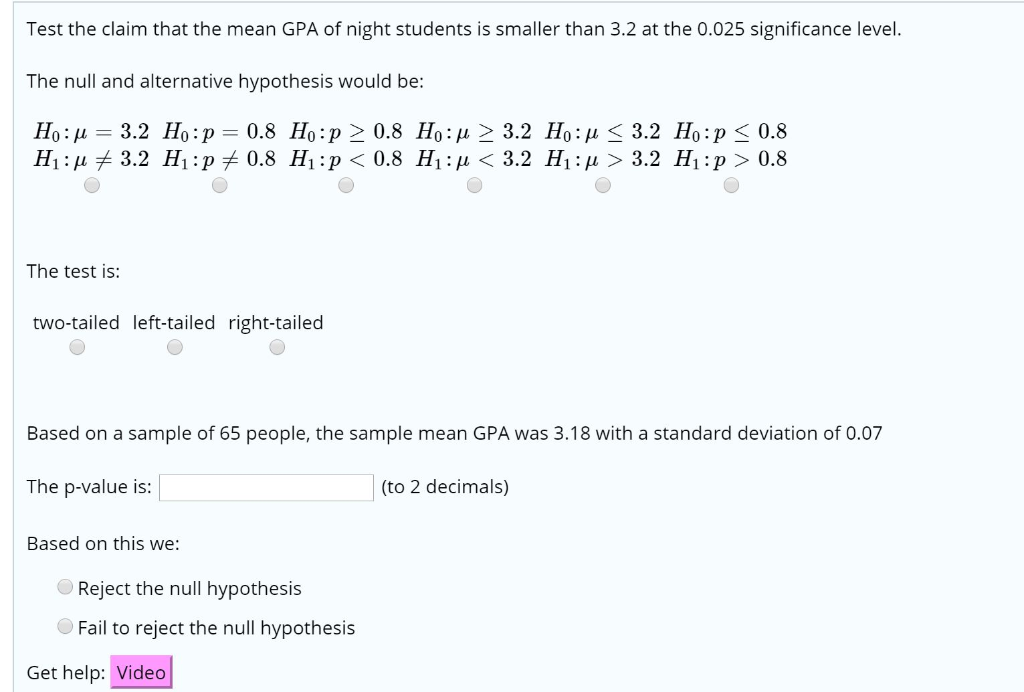Test the claim that the mean GPA of night students is smaller than 3.2 at the 0.025 significance level. The null and alternative hypothesis would be: Họ: A = 3.2 H0:p = 0.8 Ho:p> 0.8 H0:u > 3.2 H:1 < 3.2 H:p < 0.8 H1:# 3.2 H:P + 0.8 H :p < 0.8 H :p < 3.2 H1: p > 3.2 H :p > 0.8 The test is: two-tailed left-tailed right-tailed Based on a sample of 65 people, the sample...

• ### Test the claim that the mean GPA of night students is larger than 2.6 at the...

Test the claim that the mean GPA of night students is larger than 2.6 at the .10 significance level. The null and alternative hypothesis would be: H0:p=0.65H0:p=0.65 H1:p<0.65H1:p<0.65 H0:p=0.65H0:p=0.65 H1:p>0.65H1:p>0.65 H0:μ=2.6H0:μ=2.6 H1:μ<2.6H1:μ<2.6 H0:μ=2.6H0:μ=2.6 H1:μ>2.6H1:μ>2.6 H0:μ=2.6H0:μ=2.6 H1:μ≠2.6H1:μ≠2.6 H0:p=0.65H0:p=0.65 H1:p≠0.65H1:p≠0.65 The test is: left-tailed right-tailed two-tailed Based on a sample of 50 people, the sample mean GPA was 2.61 with a standard deviation of 0.02 The test statistic is:  (to 2 decimals) The critical value is:  (to 2 decimals) Based on this we: Reject...

• ### Test the claim that the mean GPA of night students is smaller than the mean GPA...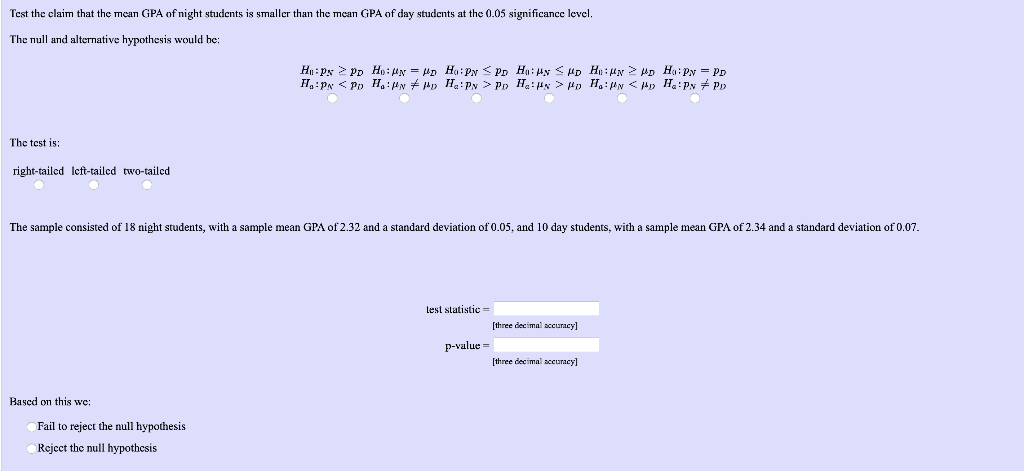Test the claim that the mean GPA of night students is smaller than the mean GPA of day students at the 0.05 significance level, The null and alternative hypothesis would he: HPxPD Ho: Un = yd H: PN PD HH:My CMD HUN MD Hai Py = PD HIPN Po H.Mn + My H.Py > Po H. x > Hp H.: 4n <H H.:Py + P The test is: right-tailed left-tailed two-tailed The sample consisted of 18 night students, with a...

• ### Test the claim that the mean GPA of night students is larger than 2.6 at the...

Test the claim that the mean GPA of night students is larger than 2.6 at the 0.10 significance level. The null and alternative hypothesis would be: H0:μ≥2.6H0:μ≥2.6 H1:μ<2.6H1:μ<2.6 H0:p≥0.65H0:p≥0.65 H1:p<0.65H1:p<0.65 H0:μ≤2.6H0:μ≤2.6 H1:μ>2.6H1:μ>2.6 H0:p=0.65H0:p=0.65 H1:p≠0.65H1:p≠0.65 H0:μ=2.6H0:μ=2.6 H1:μ≠2.6H1:μ≠2.6 H0:p≤0.65H0:p≤0.65 H1:p>0.65H1:p>0.65 The test is: two-tailed left-tailed right-tailed Based on a sample of 45 people, the sample mean GPA was 2.63 with a standard deviation of 0.07 The p-value is:  (to 2 decimals) Based on this we: Fail to reject the null hypothesis Reject the...

• ### Test the claim that the mean GPA of night students is significantly different than the mean...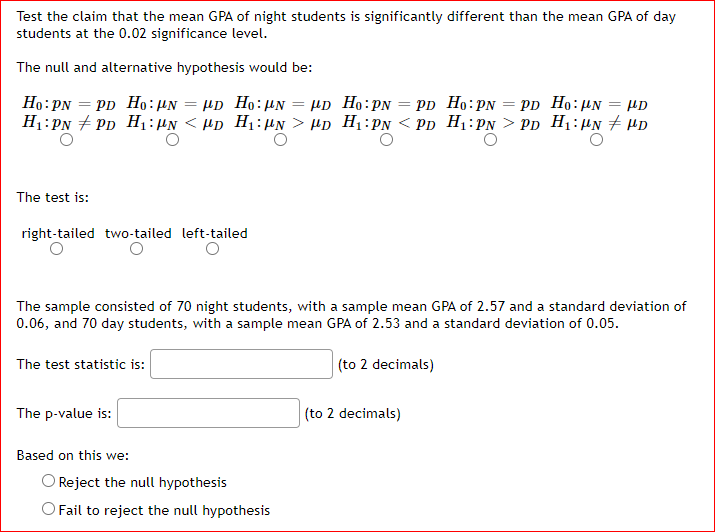Test the claim that the mean GPA of night students is significantly different than the mean GPA of day students at the 0.02 significance level. The null and alternative hypothesis would be: Ho: PN PD Ho: un up Ho:un = up Ho:PN = pd Ho: PN PD Ho: un μD H:PN + PD H: Un <Hp H: Un > Hp H:PN <PD H:PN > PD Hunt up O O The test is: right-tailed two-tailed left-tailed The sample consisted of 70...

• ### Test the claim that the mean GPA of Orange Coast students is smaller than the mean...

Test the claim that the mean GPA of Orange Coast students is smaller than the mean GPA of Coastline students at the 0.05 significance level. The null and alternative hypothesis would be: H0:pO≥pCH0:pO≥pC H1:pO<pCH1:pO<pC H0:pO≤pCH0:pO≤pC H1:pO>pCH1:pO>pC H0:μO≤μCH0:μO≤μC H1:μO>μCH1:μO>μC H0:μO=μCH0:μO=μC H1:μO≠μCH1:μO≠μC H0:μO≥μCH0:μO≥μC H1:μO<μCH1:μO<μC H0:pO=pCH0:pO=pC H1:pO≠pCH1:pO≠pC The test is: right-tailed two-tailed left-tailed The sample consisted of 55 Orange Coast students, with a sample mean GPA of 2.69 and a standard deviation of 0.05, and 55 Coastline students, with a sample mean GPA...

• ### Test the claim that the mean GPA of Orange Coast students is smaller than the mean...

Test the claim that the mean GPA of Orange Coast students is smaller than the mean GPA of Coastline students at the 0.05 significance level. The null and alternative hypothesis would be: H0:pO≥pCH0:pO≥pC H1:pO<pCH1:pO<pC H0:pO≤pCH0:pO≤pC H1:pO>pCH1:pO>pC H0:μO≤μCH0:μO≤μC H1:μO>μCH1:μO>μC H0:μO=μCH0:μO=μC H1:μO≠μCH1:μO≠μC H0:μO≥μCH0:μO≥μC H1:μO<μCH1:μO<μC H0:pO=pCH0:pO=pC H1:pO≠pCH1:pO≠pC The test is: right-tailed two-tailed left-tailed The sample consisted of 55 Orange Coast students, with a sample mean GPA of 2.69 and a standard deviation of 0.05, and 55 Coastline students, with a sample mean GPA...